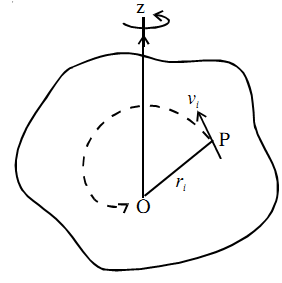The product of linear momentum and the distance from the axis is called angular momentum, denoted by L.L = ∑ mi ω ri ri = (Σ mi ri2

L = Iω

The angular velocity is the same for all the particles and the term within brackets is the moment of inertia. Like the linear momentum, the angular momentum is also a vector quantity. The unit of angular momentum is kg m2 s–1.

The rate of change of ω is α and Iα = τ. Therefore, the rate of change of angular momentum is equal to torque.

dL/dt = τ = I dω/dt = I α